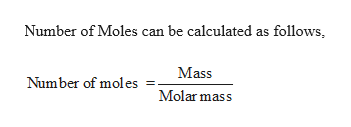# Calculate the number of moles in each sample.74.9 g H2CCl2 n(H2CCl2)=??

Question
105 views

Calculate the number of moles in each sample.

74.9 g H2CCl2

n(H2CCl2)=??

check_circle

Step 1

Given,

74.9 g of dich...help_outlineImage TranscriptioncloseNumber of Moles can be calculated as follows Mass Number of mol es Molar mass fullscreen

### Want to see the full answer?

See Solution

#### Want to see this answer and more?

Solutions are written by subject experts who are available 24/7. Questions are typically answered within 1 hour.*

See Solution
*Response times may vary by subject and question.
Tagged in